## Introduction

The integration of a SOFC in a gas turbine cycle brings the advantage of an extremely high electrical efficiency that cannot be achieved by a gas turbine alone. In particular, efficiency on coal syngas could be competitive with combined cycles, and, especially for small size plants, this type of system promises the highest conversion efficiencies 06, 12, 10, which translates to reduced emissions and noteworthy economic benefits.

Compared to a standalone fuel cell, hybridization with a gas turbine introduces additional opportunities. Gas turbine capability for quick load following can improve system flexibility 07, 02; furthermore, degradation phenomena in the fuel cell can be mitigated by shifting the power production from the fuel cell to the turbine 13.

##### Table 3.

Economic assumptions.

ComponentCost
SOFC stack400 $/kW for a stack size of 330 kW 14 Gas turbine700$/kW (Rosa do Nascimento et al.)
Exhaust gas recuperator50% turbine cost (Rosa do Nascimento et al.)
SOFC blower10% stack cost 01
SOFC inverter10% stack cost 01
Annual maintenance3% capital investment
Electricity price (feed-in tariff)0.14 $/kWh Fuel price0.1$/kg
Discount rate (to actualize cash flows)0.01

In addition to these costs, a complete replacement of the stack was considered when necessary. In particular, for the standalone stack, the end of life meant the failure of the stack (when the voltage dropped zero). In the hybrid system, the stack was replaced when the turbine reached design power condition, because total system power could not be maintained constant after that point unless increasing SOFC power.

The economic benefit was evaluated in terms of PBP, NPV at 20 years, and Internal Rate of Return (IRR), calculated according to Equations 15–18. Hence, such parameters included as many fuel cell stack replacements as necessary.

TCI=j=1PBPCFNj
##### (16)
CFNj=EelPelCfPfCmain
##### (17)
NPV=j=120CFNj(1+r)jTCI
##### (18)
j=120CFNj(1+IRR)jTCI=0

## Results and comparison

First of all, for the standalone SOFC stack with constant power output, five cases were analyzed and compared. The initial current density was varied from 0.5 to 0.1 A/cm2. Consequently, the nominal power was varied from 330 to 71 kW. The stack size was assumed the same, with a design capability of 330 kW, with the advantage of extending the life of the fuel cell and reducing the number of replacements when the power density is kept lower. Obviously, there was expected to be a trade-off between sold electricity and fuel cell lifetime. FU was kept 80% in all the case studies. Figure 3 presents stack power over time in the five cases.

##### Figure 3.

Stack power over time for the standalone fuel cell at different initial current densities.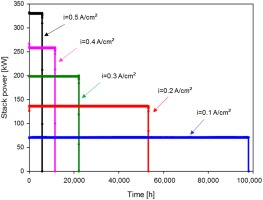As shown in Figure 3, the benefit of decreasing the power density in terms of fuel cell lifetime was substantial. Initial degradation rate was reduced from almost 1% per 1,000 h of the first case to 0.13%/1,000 h. Stack efficiency over time is presented in Figure 4.

##### Figure 4.

Efficiency trend over time for the standalone stack at different initial current densities.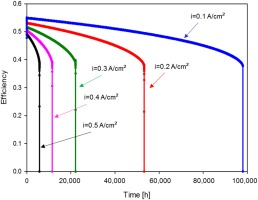In terms of PBP, reducing the power density had the negative effect of decrementing the annual cashflow (CFN) and the positive consequence of replacing the stack less times over the plant lifetime. For example, in the case with lowest current density, the stack should be replaced once every 11 years. Hence, the cost of the stack would have a great impact in determining the most beneficial strategy.

With a stack cost of 132,000 $, the case at highest current density was not economically feasible, since the cashflows were negative throughout the system lifetime. Table 4 shows PBP, NPV, and IRR, where the case at 0.2 A/cm2 appeared to be the most economically advantageous. Further reducing the power density, PBP started increasing again, indicating that there is an optimal value of current from an economic point of view. ##### Table 4. PBP, NPV, and IRR for the standalone stack at constant power with different initial current densities. Initial current density [A/cm2]Lifetime [yr]PBP [yr]NPV [% capital cost]IRR 0.50.7−538−0.075 0.41.326.5−1.50.165 0.32.57.3114.20.188 0.266.31810.214 0.111.211.263.80.106 If the stack cost increased to 1,000$/kW at 0.5 A/cm2, for a total cost of 330,000 $, only the two cases at lowest current density gave a positive PBP, equal to 53 years for 0.2 A/cm2 and 91 years for 0.1 A/cm2. In that scenario, NPV at 20 years would be negative, respectively −74% and −78% of the initial capital cost (which is now approximately three times higher). A current stack price of 3,000$/kW, which brought the total cost to 990,000 $, did not give positive PBP or NPV for any current density. In the hybrid system, stack power was reduced due to the decrement in stack current to keep constant voltage. At the same time, turbine power increased, as shown in Figure 5 where hybrid system and two standalone cases are compared. Stack lifetime was longer in the hybrid system configuration compared to the optimum standalone configuration. ##### Figure 5. Comparison of system power over time in the hybrid system and two standalone cases.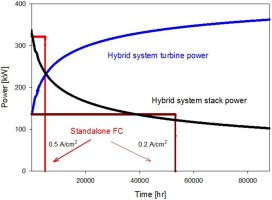Figure 6 presents FU trend over time in the two systems. FU was calculated as the ratio between consumed fuel (in terms of H2 and CO molar rates) and inlet fuel molar rate. In the standalone stack, FU was kept constant in every case to avoid fuel starvation when the current increased. In the hybrid system, FU decreased as consequence of the decreasing current and increasing fuel flow. This operating strategy brought additional benefits to stack performance, as previously discussed. ##### Figure 6. Fuel utilization over time in the hybrid system and standalone stack.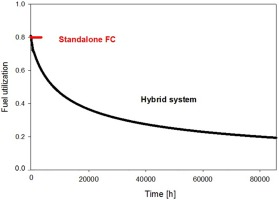In Figure 7, system efficiency is compared for the two configurations. Hybrid system efficiency is the contribution of SOFC and gas turbine efficiencies. The latter was fairly low at the beginning of plant lifetime because the turbine worked in off-design conditions, and it gradually increased over time offsetting SOFC efficiency decay. For this reason, the global efficiency was maintained almost constant over the entire time. The great difference compared with the efficiency drop in the standalone stack resulted in better economic performance. ##### Figure 7. Efficiency comparison in the hybrid system and two standalone cases.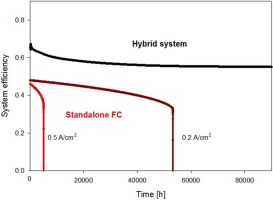The results for the hybrid system are shown in Table 5. The economic benefit compared to the standalone stack was evident. In particular, in the hybrid system, the results were not significantly affected by the stack cost. This was because the stack was replaced only once every 11 years and because of the gas turbine contribution on total power production and total system efficiency. ##### Table 5. PBP, NPV, and IRR for the hybrid system. Stack costStack lifetime [yr]PBP [yr]NPV [% capital cost]IRR 400$/kW2.94160.33
1,000 $/kW11.33.33650.29 3,000$/kW5.21890.21

This simplified analysis showed how, with these assumptions, the PBP would be halved in a hybrid configuration with respect to the most advantageous standalone case. The very low dependence on stack cost indicates that an early adoption of SOFC technology would be possible at current cost and voltage degradation rate thanks to the hybridization with gas turbine systems. Figure 8 illustrates the dependence of NPV on current density and stack cost for the standalone SOFC and the hybrid system.

##### Figure 8.

NPV trends for standalone SOFC and hybrid system with different stack costs.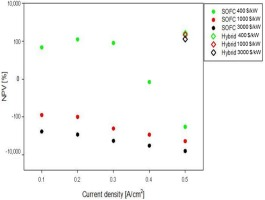## Conclusions

In this work, the impact of fuel cell degradation on the economics of the system was analyzed comparing a standalone SOFC and an advanced hybrid plant where SOFC was coupled with a gas turbine. The integration of these two different technologies favored a high system efficiency over time despite fuel cell performance degradation. The flexibility added by the gas turbine allowed an operating strategy that extended fuel cell lifetime and improved system performance. The economic results showed that the PBP for the hybrid system was half and NPV doubled compared to the SOFC best-case scenario, while the IRR increased by 54%.

In the standalone SOFC system, operating life could be extended oversizing the stack at constant power demand, which made economic results extremely dependent on stack cost. An optimal value for operating current density was found around 0.2 A/cm2. In contrast, in a hybrid system, part of the power could be produced by the most reliable component, varying the power share between fuel cell and turbine as the first one degraded. This fortunate integration doubled SOFC lifetime compared to the standalone best case and significantly improved economic results with no substantial dependence on the stack cost. Hence, hybridization with a gas turbine could facilitate early market penetration of SOFCs, at current costs and performance degradation rates. As such, the higher initial capital investment would be compensated by higher efficiency and less frequent replacements of the stack.

## NOMENCLATURE

CFN

Annual cash flow [$] FU Fuel utilization factor NPV Net present value [$]

OGB

Off-gas burner

PBP

Pay-back period [yr]

SOFC

Solid oxide fuel cell

TCI

Total capital investment [$] TPB Triple phase boundary Cf Fuel consumption [kg] Cmain Maintenance cost [$]

cp

specific heat [J/kg K]

Eel

Electricity production [kWh]

F

G

Gibbs free energy [kJ]

H

specific enthalpy variation from 298 K [kJ/kg]

i

current density [A/cm2]

i0

exchange current density [A/cm2]

IRR

Internal Rate of Return

m˙

Mass flow rate [kg/s]

n

number of transferred electrons

n˙

molar rate [mol/s]

Pel

Electricity price [$/kWh] Pf Fuel price [$/kg]

PGT

Gas turbine power [kW]

p

pressure [bar]

Q˙

Fuel cell thermal output [kW]

R

Area specific resistance [Ω m2]

Rg

Ideal gas constant [J/mol K]

rd

T

Temperature [K]

t

time [h]

V

Voltage, overpotential [V]

x

mole fraction

α

charge transfer coefficient

η

efficiency

ν

stoichiometric coefficient

act

activation

deg